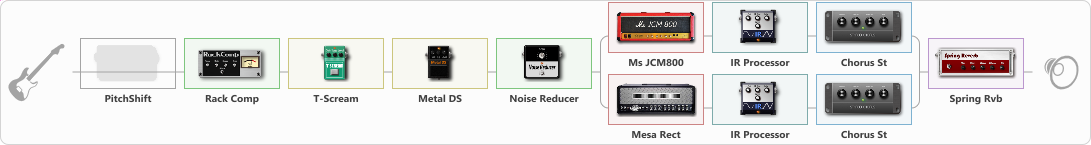# JCM 800 + Mesa Rect for rhythm guitar

Discussion in 'ToneLib-GFX presets' started by Summers, Mar 22, 2023.

1. JCM 800 + Mesa Rect for rhythm guitar

Preset name: JCM 800 + rectifier for rhythm guitar

fix noise gate, and tweak some eq, then you're ready to riff

Effects chain:Effect: "PitchShift" (Modulation / Sfx), active - "no"
{
"Pitch" = -1
"Fine" = 0
"Tracking" = 40
"Direct" = 0
"Effect" = 100
}

Effect: "Rack Comp" (Dynamics / Filter), active - "yes"
{
"Threshold (dB)" = -20
"Ratio" = 3
"Attack" = Fast
"Release (ms)" = 42
"Knee" = 49
"Level (dB)" = 0
}

Effect: "T-Scream" (Overdrive / Distortion), active - "yes"
{
"Drive" = 0
"Tone" = 100
"Level" = 100
}

Effect: "Metal DS" (Overdrive / Distortion), active - "yes"
{
"Dist" = 52
"Bass" = 56
"Middle" = 60
"Treble" = 46
"Level" = 57
}

Effect: "Noise Reducer" (Dynamics / Filter), active - "yes"
{
"Sens" = 63
"Mode" = Hard
}

Effect: "Splitter" (Dynamics / Filter)
{
"A-Bypass" = Off
"A-Pan" = -25
"A-Level" = 55
"B-Bypass" = Off
"B-Pan" = 25
"B-Level" = 55
"Width" = 0

'A' branch:
{

Effect: "Ms JCM800" (Amp simulators), active - "yes"
{
"Gain" = 81
"Bass" = 40
"Middle" = 69
"Treble" = 70
"Presence" = 67
"Master" = 69
"Level (dB)" = 2
}

Effect: "IR Processor" (Cabinets), active - "yes"
{
"IR" = Brohymn-Mesa-4x12-SM57-V30-5
"Low Cut (Hz)" = 0
"Hi Cut (kHz)" = 20.0
"Mix" = 100
"Level (dB)" = 0
}

Effect: "Chorus St" (Modulation / Sfx), active - "yes"
{
"Speed" = 1.9
"Depth" = 31
"Center" = 4.4
"Mix" = 29
}
}
'B' branch:
{

Effect: "Mesa Rect" (Amp simulators), active - "yes"
{
"Gain" = 66
"Bass" = 38
"Middle" = 0
"Treble" = 65
"Presence" = 70
"Master" = 77
"Level (dB)" = 2
}

Effect: "IR Processor" (Cabinets), active - "yes"
{
"IR" = Brohymn-Mesa-4x12-SM57-V30-5
"Low Cut (Hz)" = 0
"Hi Cut (kHz)" = 20.0
"Mix" = 100
"Level (dB)" = 0
}

Effect: "Chorus St" (Modulation / Sfx), active - "yes"
{
"Speed" = 1.9
"Depth" = 31
"Center" = 4.4
"Mix" = 29
}
}
}

Effect: "Spring Rvb" (Reverberation), active - "yes"
{
"Time" = 5.1
"PreDelay" = 0
"LoDamp" = 16
"HiDamp" = 26
"Mix" = 31
}

Note: You will need to download and install the ToneLib-GFX software to use the preset.